Anzeige

# GraphTransformations.pptx

4. Jan 2023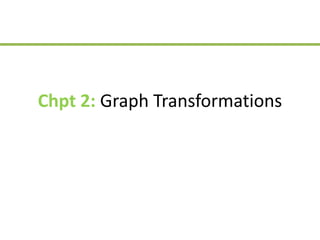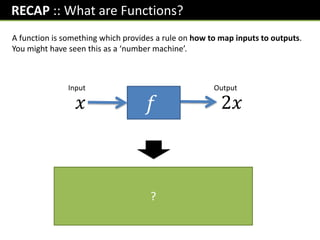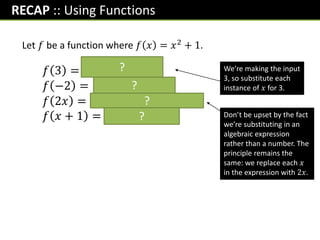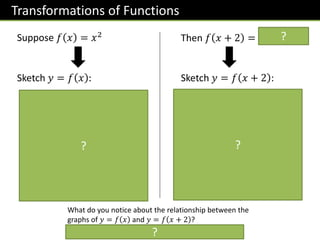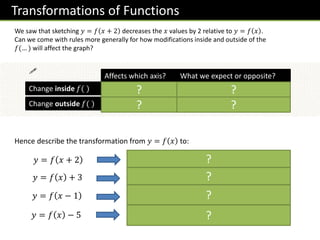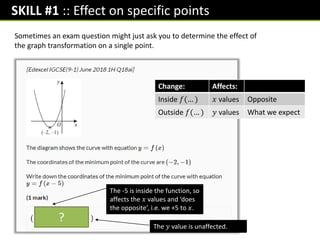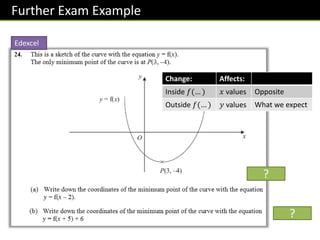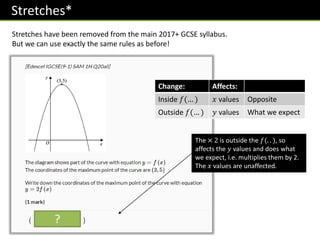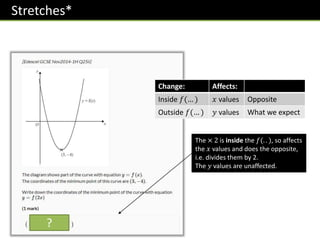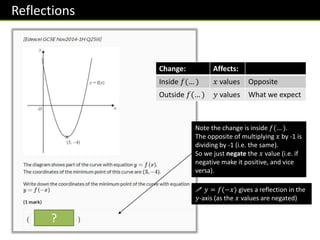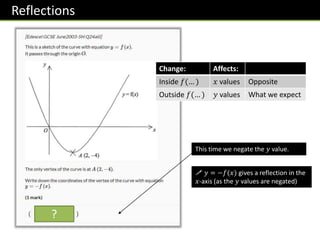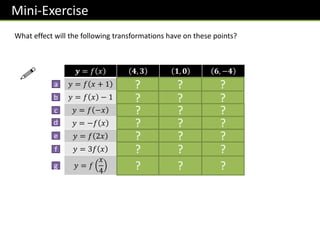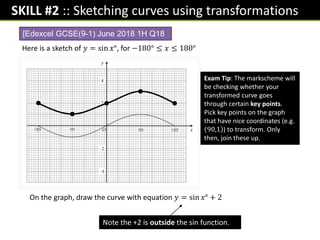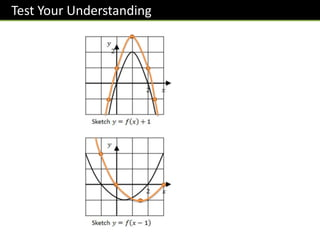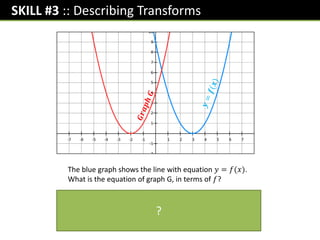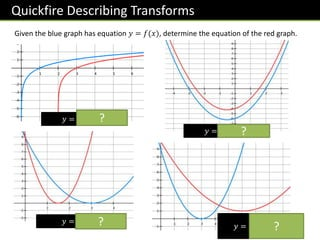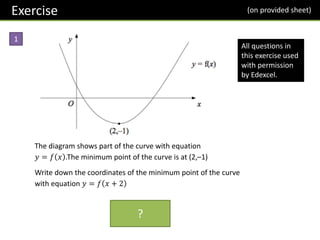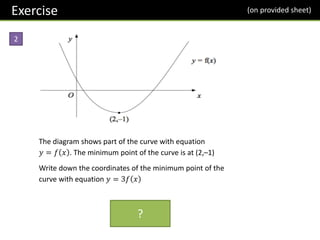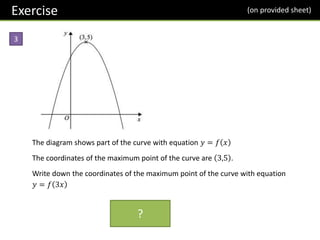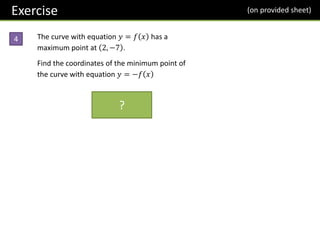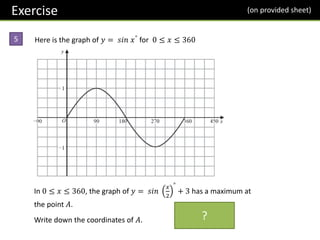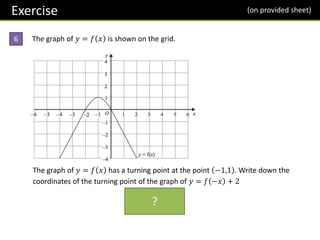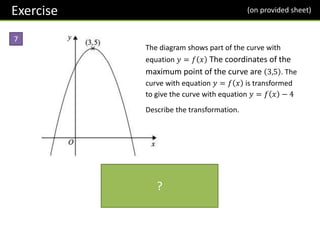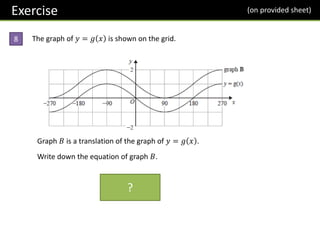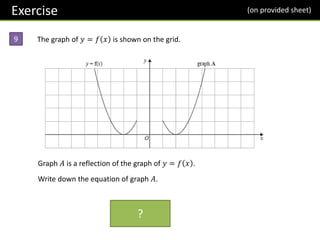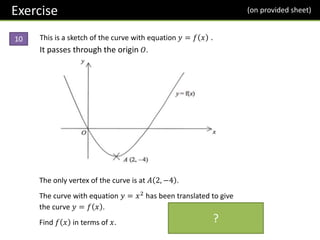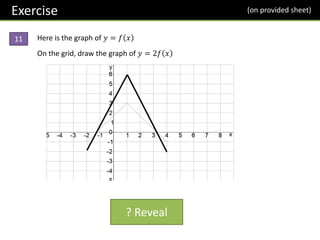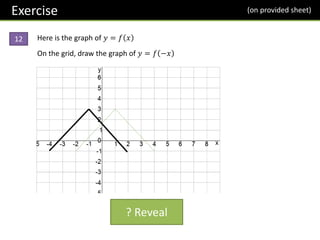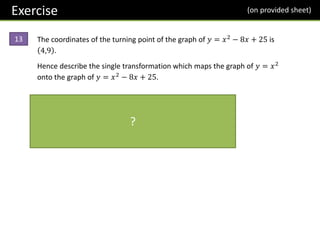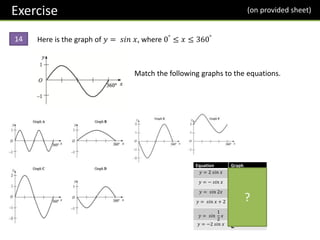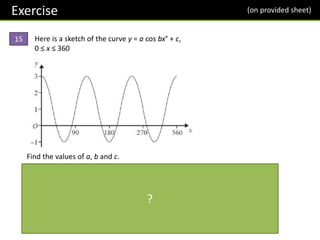1 von 32
Anzeige

### GraphTransformations.pptx

1. Chpt 2: Graph Transformations
2. www.drfrostmaths.com Everything is completely free. Why not register? Teaching videos with topic tests to check understanding. Register now to interactively practise questions on this topic, including past paper questions and extension questions (including UKMT). Teachers: you can create student accounts (or students can register themselves), to set work, monitor progress and even create worksheets. Questions organised by topic, difficulty and past paper. Dashboard with points, trophies, notifications and student progress. With questions by:
3. RECAP :: What are Functions? 𝑓(𝑥) = 2𝑥 𝑓 𝑥 2𝑥 Input Output A function is something which provides a rule on how to map inputs to outputs. You might have seen this as a ‘number machine’. Input Output Name of the function (usually 𝑓 or 𝑔) ?
4. RECAP :: Using Functions Let 𝑓 be a function where 𝑓 𝑥 = 𝑥2 + 1. 𝑓 3 = 𝟑𝟐 + 𝟏 = 𝟏𝟎 𝑓 −2 = −𝟐 𝟐 + 𝟏 = 𝟓 𝑓 2𝑥 = 𝟐𝒙 𝟐 + 𝟏 = 𝟒𝒙𝟐 + 𝟏 𝑓 𝑥 + 1 = 𝒙 + 𝟏 𝟐 + 𝟏 We’re making the input 3, so substitute each instance of 𝑥 for 3. Don’t be upset by the fact we’re substituting in an algebraic expression rather than a number. The principle remains the same: we replace each 𝑥 in the expression with 2𝑥. ? ? ? ?
5. Transformations of Functions Suppose 𝑓 𝑥 = 𝑥2 Then 𝑓 𝑥 + 2 = 𝒙 + 𝟐 𝟐 Sketch 𝑦 = 𝑓 𝑥 : Sketch 𝑦 = 𝑓 𝑥 + 2 : 𝑥 𝑦 𝑥 𝑦 −2 What do you notice about the relationship between the graphs of 𝑦 = 𝑓 𝑥 and 𝑦 = 𝑓 𝑥 + 2 ? The graph/line has translated 2 units to the left. ? ? ? ?
6. Transformations of Functions We saw that sketching 𝑦 = 𝑓 𝑥 + 2 decreases the 𝑥 values by 2 relative to 𝑦 = 𝑓 𝑥 . Can we come with rules more generally for how modifications inside and outside of the 𝑓(… ) will affect the graph? Affects which axis? What we expect or opposite? Change inside 𝑓( ) Change outside 𝑓( ) 𝑥 𝑦 Opposite What we expect ! 𝑦 = 𝑓 𝑥 + 2 Translation by −2 0 ? ? ? ? Hence describe the transformation from 𝑦 = 𝑓 𝑥 to: (i.e. reduce 𝑥 values by 2) 𝑦 = 𝑓 𝑥 + 3 Translation by 0 3 (i.e. increase 𝑦 values by 3) 𝑦 = 𝑓 𝑥 − 1 Translation by 1 0 (i.e. increase 𝑥 values by 1) 𝑦 = 𝑓 𝑥 − 5 Translation by −5 0 (i.e. reduce 𝑦 values by 5) ? ? ? ?
7. SKILL #1 :: Effect on specific points Sometimes an exam question might just ask you to determine the effect of the graph transformation on a single point. Change: Affects: Inside 𝑓(… ) 𝑥 values Opposite Outside 𝑓(… ) 𝑦 values What we expect 3 -1 ? The -5 is inside the function, so affects the 𝑥 values and ‘does the opposite’, i.e. we +5 to 𝑥. The 𝑦 value is unaffected.
8. Further Exam Example (5, -4) (-2, 2) ? ? Edexcel Change: Affects: Inside 𝑓(… ) 𝑥 values Opposite Outside 𝑓(… ) 𝑦 values What we expect
9. Stretches* Stretches have been removed from the main 2017+ GCSE syllabus. But we can use exactly the same rules as before! 3 10 ? The × 2 is outside the 𝑓(. . ), so affects the 𝑦 values and does what we expect, i.e. multiplies them by 2. The 𝑥 values are unaffected. Change: Affects: Inside 𝑓(… ) 𝑥 values Opposite Outside 𝑓(… ) 𝑦 values What we expect
10. Stretches* 1.5 -4 ? The × 2 is inside the 𝑓(. . ), so affects the 𝑥 values and does the opposite, i.e. divides them by 2. The 𝑦 values are unaffected. Change: Affects: Inside 𝑓(… ) 𝑥 values Opposite Outside 𝑓(… ) 𝑦 values What we expect
11. Reflections -3 -4 ? Note the change is inside 𝑓(… ). The opposite of multiplying 𝑥 by -1 is dividing by -1 (i.e. the same). So we just negate the 𝑥 value (i.e. if negative make it positive, and vice versa). Change: Affects: Inside 𝑓(… ) 𝑥 values Opposite Outside 𝑓(… ) 𝑦 values What we expect ! 𝑦 = 𝑓(−𝑥) gives a reflection in the 𝑦-axis (as the 𝑥 values are negated)
12. Reflections 2 4 ? This time we negate the 𝑦 value. Change: Affects: Inside 𝑓(… ) 𝑥 values Opposite Outside 𝑓(… ) 𝑦 values What we expect ! 𝑦 = −𝑓(𝑥) gives a reflection in the 𝑥-axis (as the 𝑦 values are negated)
13. Mini-Exercise What effect will the following transformations have on these points? 𝒚 = 𝑓 𝑥 𝟒, 𝟑 𝟏, 𝟎 𝟔, −𝟒 𝑦 = 𝑓 𝑥 + 1 3,3 0,0 5, −4 𝑦 = 𝑓 𝑥 − 1 4,2 1, −1 6, −5 𝑦 = 𝑓 −𝑥 −4,3 −1,0 −6, −4 𝑦 = −𝑓 𝑥 4, −3 1,0 6,4 𝑦 = 𝑓 2𝑥 2,3 0.5, 0 3, −4 𝑦 = 3𝑓 𝑥 4,9 1,0 6, −12 𝑦 = 𝑓 𝑥 4 12,3 4,0 24, −4 ? ? ? ? ? ? ? ? ? ? ? ? ? ? ? ? ? ? ? ? ? ! a b c d e f g
14. Here is a sketch of 𝑦 = sin 𝑥°, for −180° ≤ 𝑥 ≤ 180° SKILL #2 :: Sketching curves using transformations On the graph, draw the curve with equation 𝑦 = sin 𝑥° + 2 Exam Tip: The markscheme will be checking whether your transformed curve goes through certain key points. Pick key points on the graph that have nice coordinates (e.g. 90,1 ) to transform. Only then, join these up. [Edexcel GCSE(9-1) June 2018 1H Q18 Note the +2 is outside the sin function.
16. SKILL #3 :: Describing Transforms The blue graph shows the line with equation 𝑦 = 𝑓(𝑥). What is the equation of graph G, in terms of 𝑓? The graph has translated 5 units to the left. This has affected the 𝐱 values, so we do the change inside the function and do the opposite, i.e. +5 to 𝒙: 𝒚 = 𝒇(𝒙 + 𝟓) ?
17. Quickfire Describing Transforms Given the blue graph has equation 𝑦 = 𝑓(𝑥), determine the equation of the red graph. 𝑦 = 𝑓(𝑥 − 2) 𝑦 = 𝑓 𝑥 + 2 𝑦 = 𝑓(2𝑥) 𝑦 = 𝑓 1 2 𝑥 + 1 ? ? ? ?
18. Exercise (on provided sheet) 1 The diagram shows part of the curve with equation 𝑦 = 𝑓 𝑥 .The minimum point of the curve is at (2,–1) Write down the coordinates of the minimum point of the curve with equation 𝑦 = 𝑓 𝑥 + 2 𝟎, −𝟏 ? All questions in this exercise used with permission by Edexcel.
19. Exercise (on provided sheet) 2 The diagram shows part of the curve with equation 𝑦 = 𝑓 𝑥 . The minimum point of the curve is at (2,–1) Write down the coordinates of the minimum point of the curve with equation 𝑦 = 3𝑓 𝑥 (𝟐, −𝟑) ?
20. Exercise (on provided sheet) 3 The diagram shows part of the curve with equation 𝑦 = 𝑓 𝑥 The coordinates of the maximum point of the curve are 3,5 . Write down the coordinates of the maximum point of the curve with equation 𝑦 = 𝑓 3𝑥 𝟏, 𝟓 ?
21. Exercise (on provided sheet) 4 The curve with equation 𝑦 = 𝑓 𝑥 has a maximum point at 2, −7 . Find the coordinates of the minimum point of the curve with equation 𝑦 = −𝑓 𝑥 𝟐, 𝟕?
22. Exercise (on provided sheet) 5 Here is the graph of 𝑦 = 𝑠𝑖𝑛 𝑥° for 0 ≤ 𝑥 ≤ 360 In 0 ≤ 𝑥 ≤ 360, the graph of 𝑦 = 𝑠𝑖𝑛 𝑥 2 ° + 3 has a maximum at the point 𝐴. Write down the coordinates of 𝐴. 𝟏𝟖𝟎, 𝟒 ?
23. Exercise (on provided sheet) 6 The graph of 𝑦 = 𝑓 𝑥 is shown on the grid. The graph of 𝑦 = 𝑓 𝑥 has a turning point at the point −1,1 . Write down the coordinates of the turning point of the graph of 𝑦 = 𝑓 −𝑥 + 2 (𝟏, 𝟑) ?
24. Exercise (on provided sheet) 7 The diagram shows part of the curve with equation 𝑦 = 𝑓 𝑥 The coordinates of the maximum point of the curve are 3,5 . The curve with equation 𝑦 = 𝑓 𝑥 is transformed to give the curve with equation 𝑦 = 𝑓 𝑥 − 4 Describe the transformation. Translation by 𝟎 𝟒 ?
25. Exercise (on provided sheet) 8 The graph of 𝑦 = 𝑔 𝑥 is shown on the grid. Graph 𝐵 is a translation of the graph of 𝑦 = 𝑔 𝑥 . Write down the equation of graph 𝐵. 𝒚 = 𝒈 𝒙 + 𝟏 ?
26. Exercise (on provided sheet) 9 The graph of 𝑦 = 𝑓 𝑥 is shown on the grid. Graph 𝐴 is a reflection of the graph of 𝑦 = 𝑓 𝑥 . Write down the equation of graph 𝐴. 𝒚 = 𝒇 −𝒙 ?
27. Exercise (on provided sheet) 10 This is a sketch of the curve with equation 𝑦 = 𝑓 𝑥 . It passes through the origin 𝑂. The only vertex of the curve is at 𝐴 2, −4 . The curve with equation 𝑦 = 𝑥2 has been translated to give the curve 𝑦 = 𝑓 𝑥 . Find 𝑓 𝑥 in terms of 𝑥. 𝒇 𝒙 = 𝒙 − 𝟐 𝟐 − 𝟒 ?
28. Exercise (on provided sheet) 11 Here is the graph of 𝑦 = 𝑓 𝑥 On the grid, draw the graph of 𝑦 = 2𝑓 𝑥 ? Reveal
29. Exercise (on provided sheet) 12 Here is the graph of 𝑦 = 𝑓 𝑥 On the grid, draw the graph of 𝑦 = 𝑓 −𝑥 ? Reveal
30. Exercise (on provided sheet) 13 The coordinates of the turning point of the graph of 𝑦 = 𝑥2 − 8𝑥 + 25 is 4,9 . Hence describe the single transformation which maps the graph of 𝑦 = 𝑥2 onto the graph of 𝑦 = 𝑥2 − 8𝑥 + 25. Completing the square: 𝒚 = 𝒙 − 𝟒 𝟐 + 𝟗. Therefore: Translation by 𝟒 𝟗 ?
31. Exercise (on provided sheet) 14 Here is the graph of 𝑦 = 𝑠𝑖𝑛 𝑥, where 0° ≤ 𝑥 ≤ 360° Match the following graphs to the equations. Equation Graph 𝑦 = 2 𝑠𝑖𝑛 𝑥 C 𝑦 = − 𝑠𝑖𝑛 𝑥 D 𝑦 = 𝑠𝑖𝑛 2𝑥 A 𝑦 = 𝑠𝑖𝑛 𝑥 + 2 F 𝑦 = 𝑠𝑖𝑛 1 2 𝑥 B 𝑦 = −2 𝑠𝑖𝑛 𝑥 E ?
32. Exercise (on provided sheet) 15 Here is a sketch of the curve y = a cos bx° + c, 0 ≤ x ≤ 360 Find the values of a, b and c. The 𝒃 controls the horizontal stretch. Ordinarily a cos graph does ‘one cycle’ per 𝟑𝟔𝟎°, but above it does 3, so 𝒃 = 𝟑. Ordinarily a cos graph goes from -1 to 1 on the 𝒚-axis, i.e. a height of 2. But above the height is 4, so 𝒂 = 𝟐. But this would make 𝒚 be between -2 and 2, so we need to add 1 to 𝒚. Therefore 𝒄 = 𝟏. ?
Anzeige